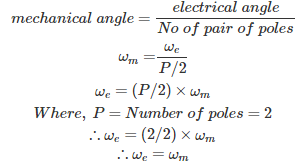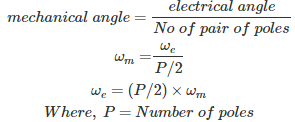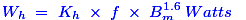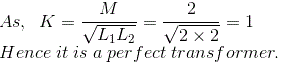# MCQs on Electrical Machines

##### Page 49 of 114. Go to page 1 2 3 4 5 6 7 8 9 10 11 12 13 14 15 16 17 18 19 20 21 22 23 24 25 26 27 28 29 30 31 32 33 34 35 36 37 38 39 40 41 42 43 44 45 46 47 48 49 50 51 52 53 54 55 56 57 58 59 60 61 62 63 64 65 66 67 68 69 70 71 72 73 74 75 76 77 78 79 80 81 82 83 84 85 86 87 88 89 90 91 92 93 94 95 96 97 98 99 100 101 102 103 104 105 106 107 108 109 110 111 112 113 114
01․ If direct axis reactance Xd and quadrature axis reactance Xq are equal, then reluctance power is
maximum
zero
minimum
all of the above

Power developed in salient pole machine contains two components. 1. Excitation power 2. Power due to saliency or reluctance Power developed in salient pole machine P = EV/Xd * sinδ + V²/2( (Xd-Xq)/(Xd*Xq)) * sin2δ Therefore, if Xd = Xq, reluctance power will become zero.

02․ A 2 pole synchronous machine is operating at 3000 rpm. Find the relation between electrical angular momentum (ωe) and mechanical angular momentum (ωm) ?
ωe = ωm
ωe = 2*ωm
ωe = 4*ωm
ωe = 1/2*ωm03․ A synchronous machine has P number of poles, find the relation between electrical angular momentum (ωe) and mechanical angular momentum (ωm)?
ωe = ωm
ωe = P*ωm
ωe = P/2*ωm
ωe = 2/P*ωm04․ Addition of 0.3 % to 4.5 % silicon to iron
increases the electrical resistivity of iron.
reduces the saturation magnetization.
increases the hysteresis loss.
none of the above.

Addition of 0.3 % to 4.5 % silicon to iron increases the electrical resistivity of iron.

05․ Hysteresis loss is proportional to
f.
f1.5.
f2.
f3.

The formula for hysteresis loss is given by,Hence, the hysteresis loss is proportional to f.

06․ A laminated iron core has reduced eddy current losses because
more wire can be used with less dc resistance in coil.
the lamination are insulated from each other.
the magnetic flux is concentrated.
the lamination are stacked vertically.

The laminations on the core are actually insulated from each other. This means that there is no circuit to make the current flow. This is how the eddy current loss is reduced by the lamination of cores which are insulated from each other.

07․ The torque speed characteristics of a AC servomotor is approximately
parabola
linearised
hyperbola
any of the above

The motors that are used in automatic control system are called as servomotors. When the objective of the system is to control the position of an object the system is called servo mechanism. The servomotors are used to convert an electrical signal applied to them into an angular displacement of the shaft. They can either operate in continuous duty or step duty depending on construction. AC servomotor construction is similar to two phase induction motor. The rotor of a AC servomotor is built with high resistance. So that its X/R ratio is small and the torque speed characteristics are approximately linearised.

08․ The two windings of a transformer have an inductor of 2 H each. If mutual inductor between them is also 2 H, then
turns ratio of the transformer is also two.
transformer is an ideal transformer.
it is a perfect transformer.
none of these.09․ DC machine is a
conduction machine.
convection machine.
both are correct.
none of above are correct.

DC machine has direct contact to conductor and controlling of current is direct. Hence it is called conduction machine.

10․ Generator works on the principle of production
dynamically induced emf.
static induced emf.
none of the above.
both A and B.

DC generator works on dynamically induced emf and AC generator works on static induced emf.

<<<4748495051>>>